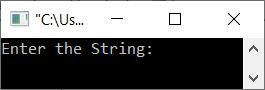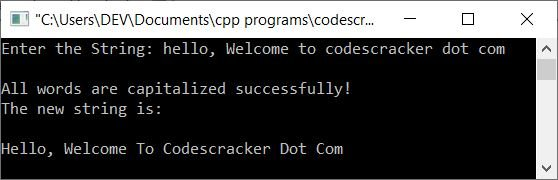# C++ Program to Capitalize the First Letter of Every Word in a String

This article shows how to write a C++ program that capitalizes the first letter of every word in a string that the user enters at run time.

For example, if the string entered by the user is "hello, welcome to codescracker dot com," then the output after capitalizing every word of the given string looks like "Hello, Welcome To Codescracker Dot Com."

## Capitalizing the first letter of all words

The question is, "Write a program in C++ that receives a string as input from the user and capitalizes every word of the given string." The answer to this question is:

```#include<iostream>
#include<string.h>
#include<stdio.h>

using namespace std;
int main()
{
char str, ch;
int len, i, asc_val;
cout<<"Enter the String: ";
gets(str);
len = strlen(str);
for(i=0; i<len; i++)
{
ch = str[i];
if(i==0)
{
asc_val = ch;
if(asc_val>=97 && asc_val<=122)
{
asc_val = asc_val-32;
ch = asc_val;
str[i] = ch;
}
}
if(ch==' ')
{
ch = str[i+1];
asc_val = ch;
if(asc_val>=97 && asc_val<=122)
{
asc_val = asc_val-32;
ch = asc_val;
str[i+1] = ch;
}
}
}
cout<<"\nAll words are capitalized successfully!";
cout<<"\nThe new string is:\n\n";
cout<<str;
cout<<endl;
return 0;
}```

Here is the initial output produced by the above C++ program on capitalizing each and every word of a given string by the user at run-time:Now supply the string input. Say "hello and welcome to codescracker.com", then press the ENTER key to capitalize all of its words, as shown in the screenshot below:From the above program, the statement:

`len = strlen(str);`

initializes the length of the string to the variable "len." And the statement:

`ch = str[i];`

initializes the character at the ith index of the string str in the variable ch. And the statement:

`asc_val = ch;`

initializes the ASCII equivalent of the character stored in the ch variable. This is because the asc_val is of the int type. Again, the statement given below

`ch = asc_val;`

initializes the character that is equivalent to the ASCII value stored in the asc_val variable to the ch variable.

Note: The ASCII value of a is 97, whereas the ASCII value of z is 122.

Note: The ASCII value of A is 65, whereas the ASCII value of Z is 90.

That is, to convert lowercase to uppercase (eg., c to C), just subtract 32 from its ASCII value. Therefore, 99 (c) - 32 = 67 (C).

C++ Quiz

« Previous Program Next Program »